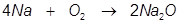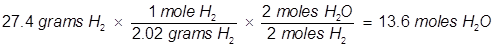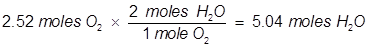# Limiting Reactants Formula

Limiting Reactants Formula

A chemical reaction will continue to run as long as there are reactants to keep it going. When one of the reactants is used up the chemical reaction stops. The reactant that is used up first is known as the limiting reactant. It is called this because it limits the amount of product that will be produced.

Identifying the limiting reactant in a chemical reaction can be done with a variety of methods. It is not enough to just look at the amounts of each reactant and pick the lesser amount. Stoichiometry plays a major role in determining which reactant truly limits.

Limiting Reactants Formula Questions:

1.Identify the limiting reactant if 5.43 moles of Na reacts with 4.25 moles of O2 in the following equation.One of the simplest ways to identify a limiting reactant is to compare how much product each reactant will produce. Stoichiometry will be used to create a ratio between reactants and products given in the balanced chemical equation.(5.43 moles of Na will produce 2.72 moles Na2O)(4.25 moles of O2 will produce 8.50 moles Na2O)

In the reaction Na will produce a lesser amount of Na2O than O2 will therefore Na is the limiting reactant. Notice that the starting amount of Na is actually larger than the starting amount of O2 but stoichiometry shows that Na will run out first.

2. Identify the limiting reactant if 27.4 grams of H2 reacts with 2.52 moles of O2 in the following equation.In this example, one of the reactant values is given in grams. It must be converted to moles in order to use the mole ratio of the balanced equation.Oxygen (O2) produces less water (H2O) than hydrogen (H2) therefore oxygen is the limiting reactant.